## Ramp Function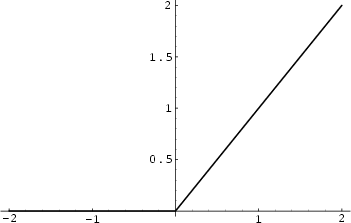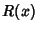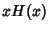(1)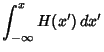(2)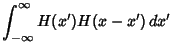(3)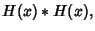(4)

where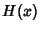is the Heaviside Step Function and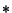is the Convolution. The Derivative is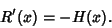(5)

The Fourier Transform of the ramp function is given by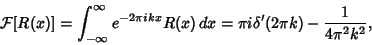(6)

where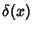is the Delta Function and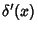its Derivative.

See also Fourier Transform--Ramp Function, Heaviside Step Function, Rectangle Function, Sgn, Square Wave